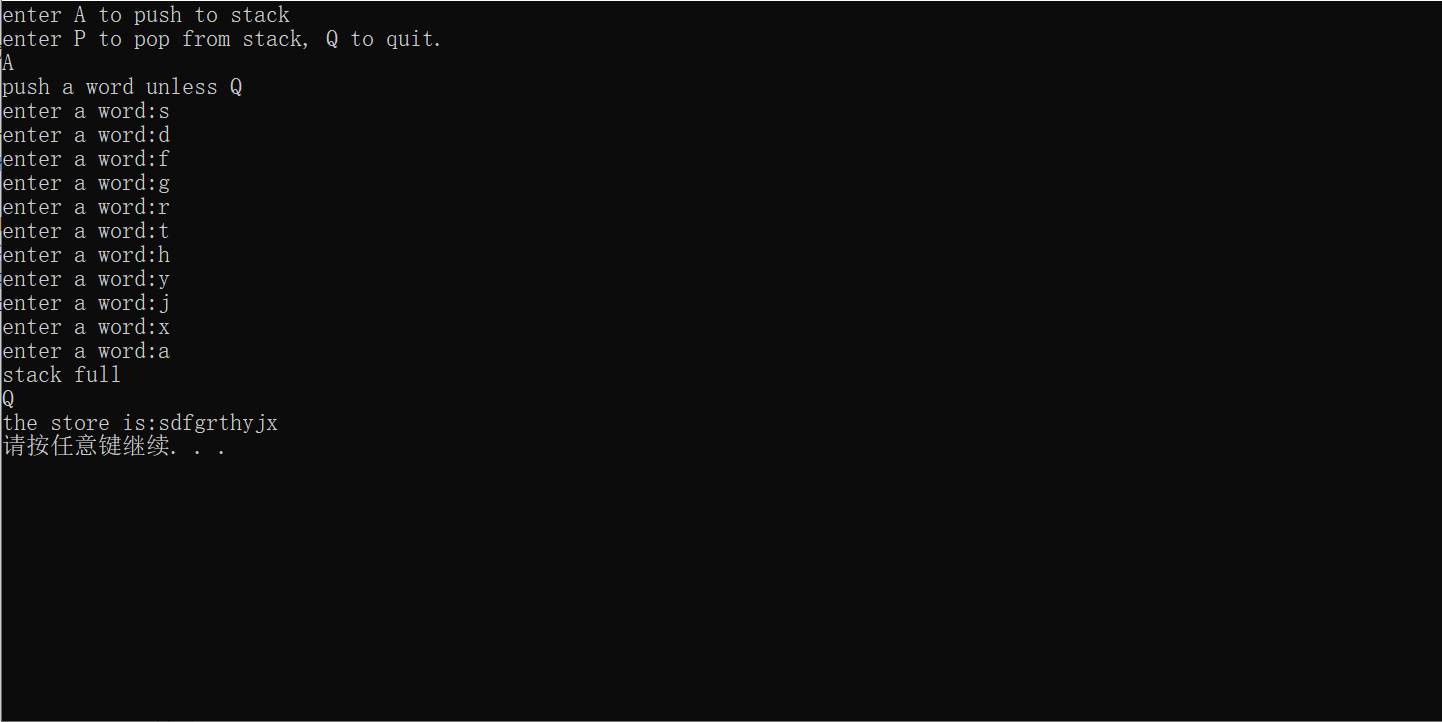﻿ C++基础（C++Primer学习）

### C++基础（C++Primer学习）

2021-12-5 18:40:42

## C++基础（五）

C++的类（继续）

``````const Stock num3("abc", 123, 212);  //创建了一个const型的对象
num3.show(); //报错
``````

``````void show() const;  //声明加上const关键字
void Stock::show() const{}  //定义函数时在括号后面加上const关键字
``````

``````Stock ob1{param1, param2,param3...};
Stock ob2={param1, param2, param3..};
``````

``````const Stock& topval(const Stock& s) const;
``````

const Stock& 表示该函数（topval)的返回值类型是一个const型的Stock类的引用。
topval(const Stock &)表示的该函数传入的参数类型必须是Stock类的引用

``````const Stock& Stock::topval(const Stock& s) const
{
if (s.total_val > total_val)
{
return s;
}
else
{
return *this;
}
}
``````

this指针的用处在这里就体现出来了，对于上面代码返回值的类型，如果返回的是该对象内的值，那么this指针就会指向调用成员的对象。

``````num1.topval(num2);  //此时的this指针指向调用的num1对象
num2.topval(num1);  //此时的this对象指向调用的num2对象
``````

``````Stock::Stock(const string &co, long sh, double share_v)
{
company = co;
shares = sh;
share_val = share_v;
set_total();
}
``````

`````` Stock  ob_list;
``````

``````Stock list = {
Stock("dd",1,2),
Stock("ss",2,3)
// 创建了两个对象
};
``````

``````Stock list = {
Stock("dd",1,2),
Stock("ss",2,3),

};
list.show();
``````

``````Stock list = {
Stock("dd",1,2),
Stock("ss",2,3),

};
``````

``````const Stock* plist = &list;
const Stock& plist1 = list;
const Stock* p = &plist->topval(list);
``````

``````Stock list = {
Stock("dd",1,2),
Stock("ss",2,3),

};
const Stock* plist = &list;
Stock& plist1 = list;
const Stock* p = &plist->topval(list);
//cout << plist;
plist1.show();
plist1.show();
list.show();
``````

``````class 类
{
private：
const int param = 10;
.....
``````

1、使用枚举来完成

``````class l类
{
private：
enum{Months = 12};
double costs[Months];
....
``````

2、使用static关键字

``````class 2类
{
private:
static const int Months = 12;
......
``````

Stock类非常具体，然而实际中常常通过定义类来表示更通用的概念。创建一个stack类来说明这种思想：

``````#pragma once
#include<iostream>

typedef unsigned long item;  //使用typedef关键字可以提高代码可移植性

class Stack
{
private:
enum{MAX = 10};
item items[MAX];
int top;
public:
Stack();
bool isempty() const;
bool isfull() const;
bool push(item& item);
bool pop(item& item);
void show();
};
``````

``````#include "stack.h"

Stack::Stack()
{
top=0;
}

bool Stack::isempty()const
{
}

bool Stack::isfull()const
{
}

bool Stack::push(item& item)
{
if (top < MAX)
{
items[top] = item;
top++;
/*l另外一种写法，更加简洁
items[++top] = item;
*/
}
else
return false;
}

bool Stack::pop(item& item)
{
if (top > 0)
{
item = items[--top];
}
else
return false;
}

void Stack::show()
{
std::cout << "the store is:";
for (int i = 0; i < MAX; i++)
{
std::cout<<items[i];
}
std::cout << '\n';
}
``````

``````Stack use_stack()
{
Stack mystack;
char ch;
char temp;
std::cout << "enter A to push to stack\n"
<< "enter P to pop from stack, Q to quit.\n";
while (std::cin >> ch && toupper(ch) != 'Q')
{
while (std::cin.get() != '\n')
continue; //用来处理回车键
switch (ch)
{
case 'A':
std::cout << "push a word unless Q" << std::endl;
while (1)
{
std::cout << "enter a word:";
std::cin >> temp;
if (temp != 'Q')
{
if (mystack.isfull())
{
std::cout << "stack full" << std::endl;
break;
}
else
mystack.push(temp);
}
else
break;
}
break;
case 'P':
if (mystack.isempty())
{
std::cout << "satck empty" << std::endl;
}
else
mystack.pop(temp);
break;
default:
continue;
}

}
mystack.show();
return mystack;
}
``````

``````int main()
{
Stack mystack=use_stack();
......
``````